# Matrix Operations

In this section, you will learn common mathematical operations performed on matrices. These operations range from common arithmetic operations such as addition, subtraction, multiplication to transpose matrices.

Before we proceed to learn about matrix operations, you should learn common matrix notations and terminologies used in this section.

### Common Matrix Notations

An augmented matrix is derived from the system of linear equations, but there are other areas where a matrix appear as two dimensional data set with rows and columns. In general,

“A matrix is rectangular arrays of numbers called entries of the matrix”

Example

\begin{aligned}
A = \begin{bmatrix} 1 & 2 & 3\\4 & 5 & 6\\7 & 8 & 9\end{bmatrix}
B = \begin{bmatrix} a_{11} & a_{12} & a_{13}\\a_{21} & a_{22} & a_{23}\\a_{31} & a_{32} & a_{33}\end{bmatrix}
\end{aligned}

The matrix itself is denoted by a capital letter such as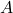or. The entries of matrices are real numbers that are denoted with lowercase letters such as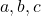etc. The subscript to the entry element denotes the position of the element within the matrix. For example,

If A is the matrix, then

\begin{aligned}
(A)_{ij} = a_{ij}
\end{aligned}

where i is row number and j is the column number.

Size of the Matrix

The size of the matrix is denoted in terms of its rows and columns. Given a matrix, the size is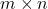where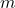is rows and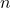is columns. You can use any letters to represent rows and columns, however, common practice is to useand.

\begin{aligned}
C_{2 \times 3} = \begin{bmatrix} a_{11} & a_{12} & a_{13}\\a_{21} & a_{22} & a_{23}\end{bmatrix}_{2 \times 3}
\end{aligned}

The shortcut notation to represent a matrix is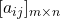or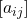.

A matrix of size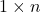is a row matrix and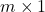is a column matrix which can be denoted using bold lowercase letters.

\begin{aligned}
&\textbf{a} = \begin{bmatrix} a_{11} & a_{12} & ... & a_{1n}\end{bmatrix}\\\\
&\textbf{c} = \begin{bmatrix} c_{11} \\ c_{12} \\ : \\ : \\ c_{m1}\end{bmatrix}
\end{aligned}

### Equality Of Matrix

How do we know if two matrices are equal?

Two matrices,andare equal i.e,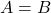if and only if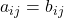.

Two matrices are equal if and only if their size are equal and all entries are equal.

Example

Let their be four matrices –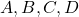.

\begin{aligned}
&A  = \begin{bmatrix} 1 & 3\\ 2 & 5\end{bmatrix}_{2 \times 2}\\\\
&B  = \begin{bmatrix} 4 & 1\\ -1 & 6\\ 3 & -1\end{bmatrix}_{3 \times 2}\\\\
&C  = \begin{bmatrix} 1 & 3\\ 2 & 5\end{bmatrix}_{2 \times 2}\\\\
&D  = \begin{bmatrix} 0 & 3\\ 7 & 5\end{bmatrix}_{2 \times 2}
\end{aligned}

Lets compare matrix A with other matrices.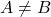because the size and elements does not match at all.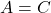because the size and elements does match perfectly.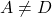because the size match but the elements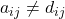for some corresponding elements.

### Common Matrix Operations

There are many elementary row operation about which you learned in the previous sections. However, we can perform some basic mathematical operations on elements of matrix as well. There are

2. Matrix Subtraction
3. Matrix Multiplication
4. Scalar Multiplication With Matrix
5. Transpose Matrix
6. Trace Of Matrix

The above are some common matrix operations you will find through linear algebra course. There are some advanced and complex operations about which we discuss in later part of the linear algebra tutorial.